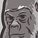# New to Qlik Sense

If you’re new to Qlik Sense, start with this Discussion Board and get up-to-speed quickly.

Announcements
Support Cases coming to Qlik Community Oct. 4! Start chats, open cases, explore resources: READ DETAILS
cancel
Showing results for
Did you mean:Contributor III

## How to get the max Month in Set analysis

Hi All,

How to get the Max Month in Set analysis

Table Format

HPA Month   Series   Value

December     123      4578

December     456      6735

November     789      8567

November     657      7894

November     876     6504

October         345      7867

Sum({<[HPA Month]={'\$(=MaxString([HPA Month]))'}>} Value)

I took the same code but I am not getting the value instead of December value I am getting the October value

I tried with all the below mentioned codes

Sum({<[HPA Month]={'\$(=MaxString([HPA Month]))'}>} Value)

Sum({<[HPA Month]={\$(=MaxString([HPA Month]))}>} Value)

Sum({<[HPA Month]={\$(=Max([HPA Month]))}>} Value)

Sum({<[HPA Month]={'\$(=Max([HPA Month]))'}>} Value)

2 Solutions

Accepted SolutionsPartner

I think the problem is that your [HPA Month] is being evaluated as text and there is no way for Qlik to know the ranking of text. You first need to convert it to a month using Date#(), evaluate it using Max() to get the highest one and then format it as a month using Date(). This should work:

Sum({<[HPA Month]={'\$(=Date(Max(Date#([HPA Month],'MMMM')),'MMMM'))'}>} Value)

Hope this helps.

Regards,

MauritzMVP

Try like:

Sum({<[HPA Month]={'\$(=Date(Max(Date#([HPA Month], 'MMMM')), 'MMMM'))'}>} Value)

2 RepliesPartner

I think the problem is that your [HPA Month] is being evaluated as text and there is no way for Qlik to know the ranking of text. You first need to convert it to a month using Date#(), evaluate it using Max() to get the highest one and then format it as a month using Date(). This should work:

Sum({<[HPA Month]={'\$(=Date(Max(Date#([HPA Month],'MMMM')),'MMMM'))'}>} Value)

Hope this helps.

Regards,

MauritzMVP

Try like:

Sum({<[HPA Month]={'\$(=Date(Max(Date#([HPA Month], 'MMMM')), 'MMMM'))'}>} Value)Tags
Community Browser